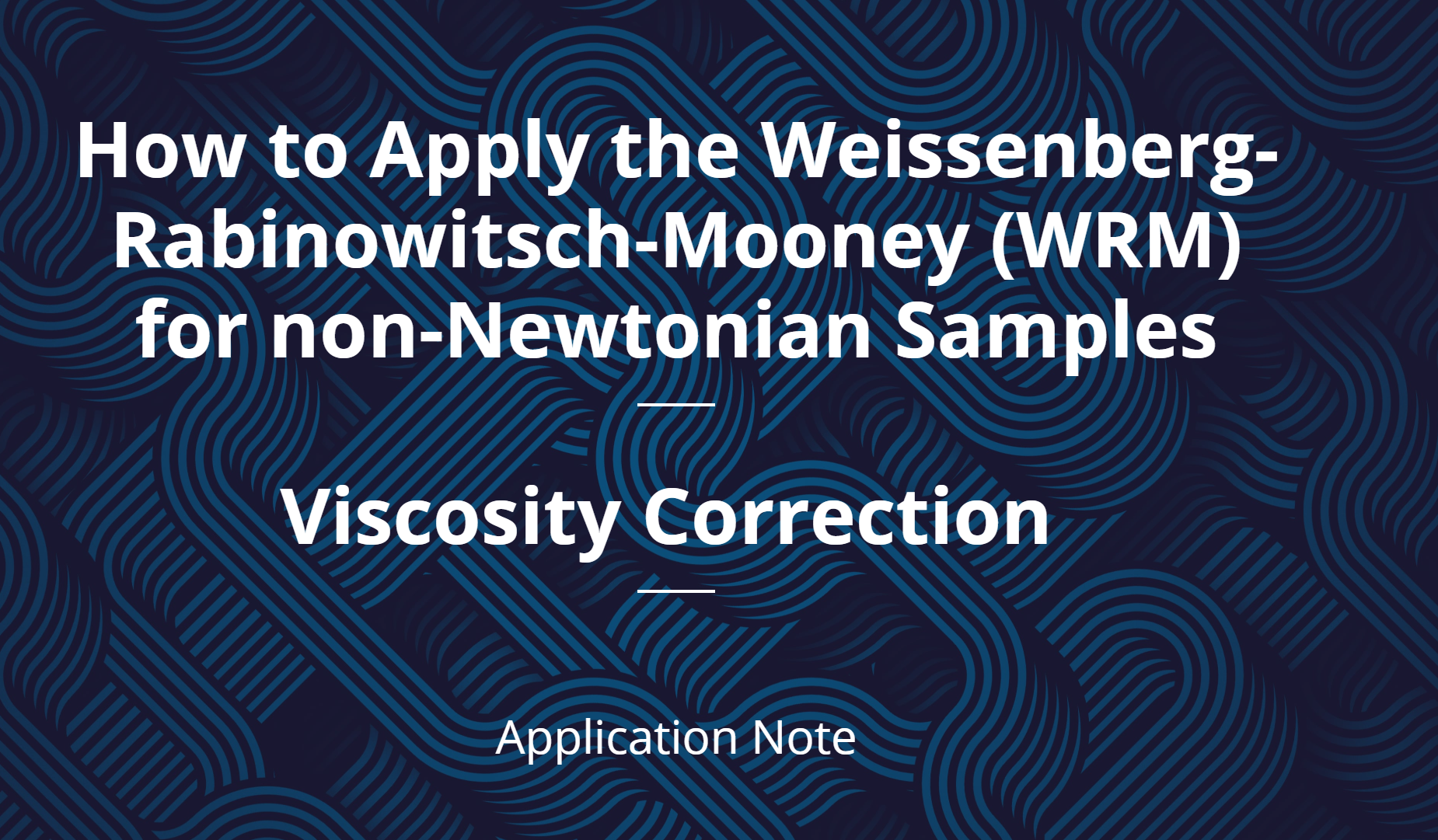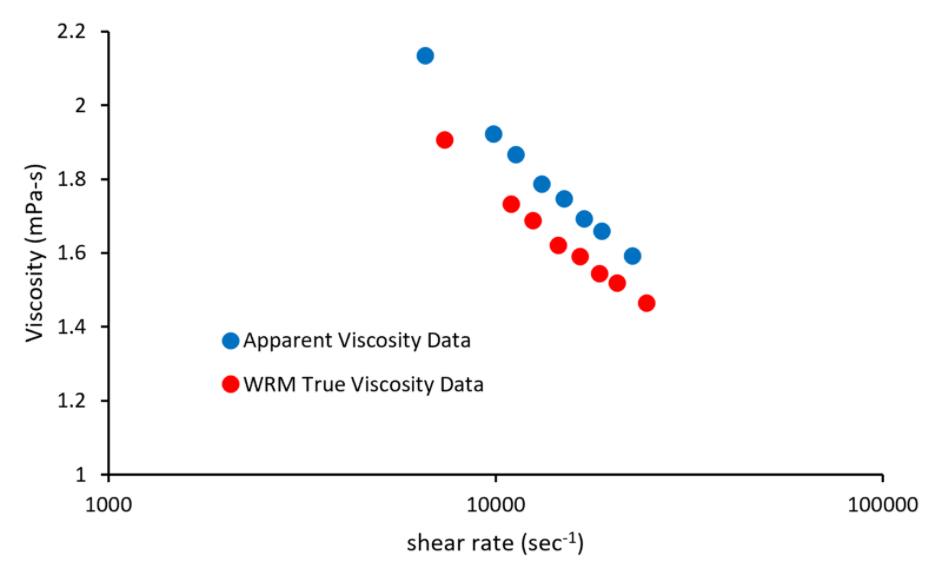# How to Apply the Correction for non-Newtonian

May 12, 2020

The vast majority of fluids in the world exhibit non-Newtonian behavior. Because of this behavior, knowing how to measure, correct, and handle non-Newtonian rheological data is vital for performing accurate and repeatable experiments and data analysis.

Knowing how to process and analyze non-Newtonian fluid data from viscometers is important when characterizing formulations across multiple fields. Non-Newtonian fluids are produced in the oil and gas, pharmaceutical, cosmetics, and chemical industries. Depending on the viscometer used to collect rheological data from non-Newtonian fluids, different correction methods must be performed to get accurate results from experiments. Non-Newtonian fluid behavior means that the viscosity of the fluid is dependent on the shear rate applied to the fluid.

Some viscometers, including rectangular slit viscometers, apply multiple shear rates across a flow field because of their geometry. Because the shear rate changes within the flow field, both the shear rate and the viscosity must be corrected once data is collected. One such correction is Weissenberg-Rabinowitsch-Mooney (WRM) analysis, which is an analysis method that can determine the “true shear rate,” using the “apparent shear rate” measured by the viscometer (Macosko, 1994). In this case, the “apparent shear rate” is the shear rate measured by the viscometer, while the “true shear rate” is the actual shear rate experienced by the non-Newtonian fluid, while flowing through the viscometer.In our new application note we will show step-by-step how to perform the commonly used WRM shear rate correction for non-Newtonian fluids. Beginning with the definition of viscosity and how VROC® technology measures pressure and calculates viscosity. We then show how to make the correction by calculating the true shear rate experienced by the non-Newtonian fluid from the apparent shear rate calculated by the viscometer. This correction will help ensure your future experiments and data analysis can be done with peace of mind!